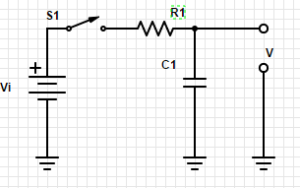1.13 RC circuits: V and I versus time

Let’s look at how voltage (V) and current (I) change with respect to time in circuits with capacitors and resistors.

Take a look at this circuit:Close the switch S1 connecting the capacitor (C1) to the battery (Vi) through resistor (R1).  How fast will C1 charge?

That is determined by the relationship between R1 and C1.  It is known as the RC time constant.

Assuming that C1 = 1 microfarad and R1 = 1k ohm, then the time constant will be 1.   And we have a shortcut that it will be within 1% of the input voltage with in 5RC, so within 5 seconds.